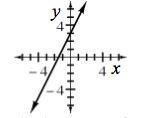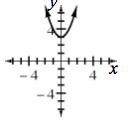### Home > INT3 > Chapter Ch1 > Lesson 1.2.2 > Problem1-92

1-92.

Graph the following functions.

Put in $y =$ form first. Make a table if you cannot remember your graphing shortcuts.

1. $y - 2x = 3$1. $y - 3 = x^2$1. State the $x$- and $y$- intercepts for each function.

$\text{a}: x\text{-intercept}=\left(-\frac{3}{2},0\right);\ y\text{-intercept} = (0, 3)$

$\text{b}:\text{ no }x\text{-intercept; }y\text{-intercept }=(0, 3)$

2. Where do the two graphs intersect? Show how you can algebraically solve for these two points.

When two graphs intersect, they are at the same $x$ and $y$ values.

$2x + 3 = x^2 + 3$